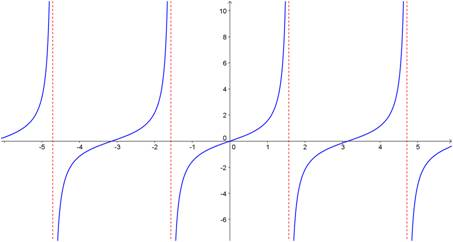## The “parent” functions

Do you know your parent functions? I’m surprised every year when some of my students don’t know how to graph some of the elementary functions they’ve learned in class. Your chances for success in precalc and calculus are significantly better if you memorize the shapes of these ten functions. No excuses, no shortcuts. Just learn them.

y = xn (Power functions)

y = |x| (absolute value function)

y = ⌊x⌋ (Greatest integer function)

y = √x

y = 1/x

y= bx (exponential function – growth and decay)

y = log x

y = sin x and y = cos x

y = tan xBlue Taste Theme created by Jabox